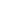FLAT 50% OFF

ENDS IN

• JEE Main & Advanced
•• 11th
•• Mathematics
• Back to Doubt Clearing

Pls solve it and explain how to do easily summation problemsFind the value of $\sum_{n=0}^{100} i^{n !}$ (where, $i = \sqrt {-1}$).

Asked By Subhash kandepi

Updated Wed, 21 Nov 2018 03:12 pm